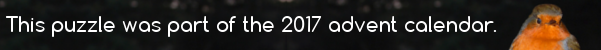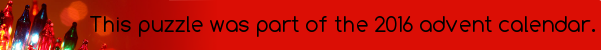mscroggs.co.uk
mscroggs.co.uksubscribe

# Puzzles

## 24 DecemberToday's number is the smallest number with exactly 28 factors (including 1 and the number itself as factors).

## 21 DecemberThe factors of 6 (excluding 6 itself) are 1, 2 and 3. $$1+2+3=6$$, so 6 is a perfect number.
Today's number is the only three digit perfect number.

## 20 DecemberWhat is the largest number that cannot be written in the form $$10a+27b$$, where $$a$$ and $$b$$ are nonnegative integers (ie $$a$$ and $$b$$ can be 0, 1, 2, 3, ...)?

## Elastic numbers

Throughout this puzzle, expressions like $$AB$$ will represent the digits of a number, not $$A$$ multiplied by $$B$$.
A two-digit number $$AB$$ is called elastic if:
1. $$A$$ and $$B$$ are both non-zero.
2. The numbers $$A0B$$, $$A00B$$, $$A000B$$, ... are all divisible by $$AB$$.
There are three elastic numbers. Can you find them?

## 16 DecemberPut the digits 1 to 9 (using each digit exactly once) in the boxes so that the sums are correct. The sums should be read left to right and top to bottom ignoring the usual order of operations. For example, 4+3×2 is 14, not 10. Today's number is the largest number than can be made from the digits in red boxes.
 × × = 6 × × × × × = 180 × × × × × = 336 =32 =70 =162

## 14 DecemberIn July, I posted the Combining Multiples puzzle.
Today's number is the largest number that cannot be written in the form $$27a+17b$$, where $$a$$ and $$b$$ are positive integers (or 0).

## 8 DecemberToday's number is the second smallest number that can be written as a×b×c×d×e×f×g×h×i, where a,b,...,i are all integers greater than 1.

## 5 DecemberToday's number is the number of ways that 35 can be written as the sum of distinct numbers, with none of the numbers in the sum being divisible by 9.
Clarification: By "numbers", I mean (strictly) positive integers. The sum of the same numbers in a different order is counted as the same sum: eg. 1+34 and 34+1 are not different sums. The trivial sum consisting of just the number 35 counts as a sum.

## Archive

Show me a random puzzle
▼ show ▼